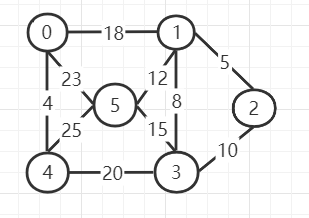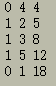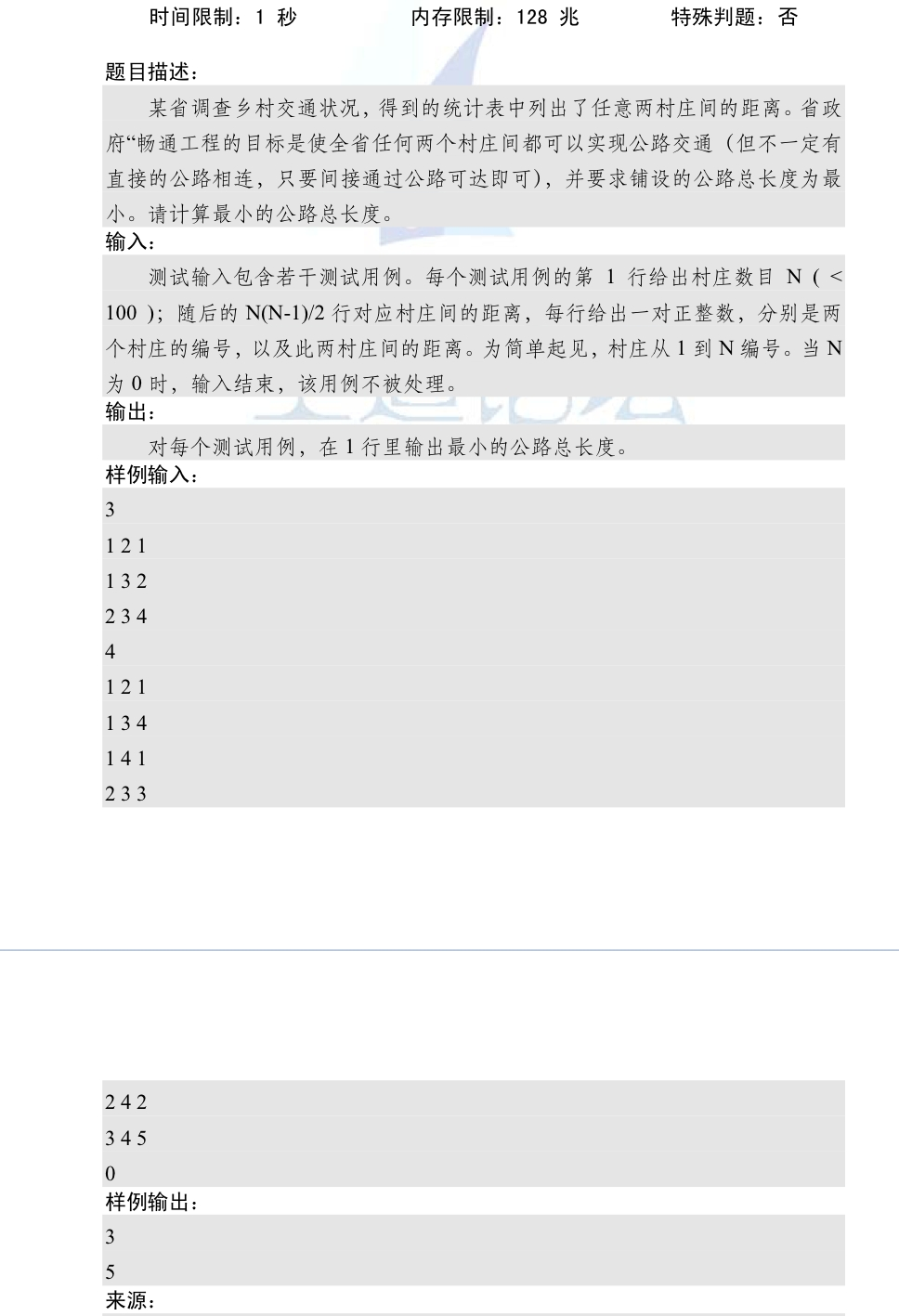• 最小生成树数模板（kruscal算法和prime算法）
千次阅读
2020-10-08 16:51:55

## kruscal算法

#include<iostream>
#include<cstdio>
#include<algorithm>
using namespace std;
int n,m,tot=0,k=0;//n端点总数，m边数，tot记录最终答案，k已经连接了多少边
int fat;//记录集体老大
struct node
{
int from,to,dis;//结构体储存边
}edge;
bool cmp(const node &a,const node &b)//sort排序（当然你也可以快排）
{
return a.dis<b.dis;
}
int father(int x)//找集体老大，并查集的一部分
{
if(fat[x]!=x)
return father(fat[x]);
else return x;
}
void unionn(int x,int y)//加入团体，并查集的一部分
{
fat[father(y)]=father(x);
}
int main()
{
scanf("%d%d",&n,&m);//输入点数，边数
for(int i=1;i<=m;i++)
{
scanf("%d%d%d",&edge[i].from,&edge[i].to,&edge[i].dis);//输入边的信息
}
for(int i=1;i<=n;i++) fat[i]=i;//自己最开始就是自己的老大 （初始化）
sort(edge+1,edge+1+m,cmp);//按权值排序（kruskal的体现）
for(int i=1;i<=m;i++)//从小到大遍历
{
if(k==n-1) break;//n个点需要n-1条边连接
if(father(edge[i].from)!=father(edge[i].to))//假如不在一个团体
{
unionn(edge[i].from,edge[i].to);//加入
tot+=edge[i].dis;//记录边权
k++;//已连接边数+1
}
}
printf("%d",tot);
return 0;
}


## prime算法

#include <bits/stdc++.h>
using namespace std;
int n, m;
int e, book;
int dis;
int inf = 0x3f3f3f3f;
int ans = 0;
int prime()
{
book = 1;
for(int i = 1; i < n; i++)
{
int minn = inf;
int u = 0;
for(int j = 1; j <= n; j++)
{
if(book[j] == 0 && dis[j] < minn)
{
minn = dis[j];
u = j;
}
}
if(u == 0)
return -1; //如果不连通返回-1
book[u] = 1;
ans += dis[u];
for(int j = 1; j <= n; j++)
{
if(book[j] == 0 && e[u][j] < dis[j])
dis[j] = e[u][j];
}
}
return ans;
}
int main()
{
cin >> n >> m;
memset(book, 0, sizeof(book));
for(int i = 1; i <= n; i++)
{
for(int j = 1; j <= n; j++)
{
if(i == j)
e[i][j] = 0;
else
e[i][j] = inf;
}
}
for(int i = 0; i < m; i++)
{
int x, y, z;
cin >> x >> y >> z;
if(z < e[x][y])  //！！！不要忘记判断！！！
e[x][y] = e[y][x] = z; //！！！不要忘记是无向图
}
for(int i = 1; i <= n; i++)
dis[i] = e[i];
int res = prime();
if(res == -1)
cout << "orz";
else
cout << res;
return 0;
}


更多相关内容
• 资源包里包含多种kruscal算法，有C语言和c++两种语言，有从文件里输入也有从程序里输入，附带报告，多的不说了。
• } cout 结果： 1、今天重新复习了一下kruscal算法，并且使用了C++复现了算法过程。 2、对于最小生成树的算法还有prim算法。 3、重点在于： （1）根据权重排序。 （2）判断新的edge的fromvex和endvex是否出现在同一...

一个无向图，寻找最小代价生成树：#include <iostream>
#include <string>
#include <vector>
#include <algorithm>
#include <set>
using namespace std;
struct edge{
int fromvex;
int endvex;
int weight;
edge(int fv,int ev,int we):fromvex(fv),endvex(ev),weight(we){}
};
bool compare(edge a,edge b)
{
return a.weight<b.weight;
}
template<typename T>
std::ostream& operator<<(std::ostream& os, const std::vector<T>& vec)
{
for (auto& el : vec)
{
os << el << ' ';
}
return os;
}
std::ostream& operator<<(std::ostream& os, const edge & vec)
{
os<<vec.fromvex<<" "<<vec.endvex<<" "<<vec.weight<<endl;
return os;
}
int Kruscal()
{
vector<edge> edgeInfo{edge(4,5,25),edge(0,5,23),
edge(3,4,20),edge(0,1,18),
edge(3,5,15),edge(1,5,12),
edge(2,3,10),edge(1,3,8),
edge(1,2,5),edge(0,4,4)};//unsorted graphic edgeset

sort(begin(edgeInfo),end(edgeInfo),compare);//  sorted graphic edgeset by weight

vector<edge> kruscal;
vector<set<int>> connectedCom;//different connected component
//init connected commponent
//Total 6 graph vertices
int vernumber = 6;
for(int i = 0;i<vernumber;i++)
{
set<int> a{i};
connectedCom.push_back(a);
}

int k = vernumber - 1;// k edge is needed in mininum cost spanning tree
int index = 0;// which edge in sorted edge
int m1 = 0,m2 = 0;
while(k>0)
{
//serach edgeInfo[index].fromvex and endvex in different connectedCom
for(size_t i = 0;i<connectedCom.size();i++)
{
for(int n:connectedCom[i])
{
if(n==edgeInfo[index].fromvex) m1 = i;
if(n==edgeInfo[index].endvex)  m2 = i;
}
}
//if fromvex endvex is in different connectedCom
if(m1!=m2)
{
kruscal.push_back(edgeInfo[index]);
k--;
for(int n:connectedCom[m1])
{
connectedCom[m2].insert(n);
}
//delete one of set<int> in connectedCom sets
connectedCom[m1].clear();
}
//next edge
index++;
}
cout<<kruscal<<endl;
return 0;
}
int main()
{
Kruscal();
return 0;
}

结果：1、今天重新复习了一下kruscal算法，并且使用了C++复现了算法过程。

2、对于最小生成树的算法还有prim算法。

3、重点在于：

（1）根据权重排序。

（2）判断新的edge的fromvex和endvex是否出现在同一个连通域里面。（每个连通域不能出现回路）

（3）每两个连通域合在一起，就要消除其中一个连通域。

展开全文• kruscal算法是用于生成最小生成树的常见算法，最小生成树也就是在包含图中所有节点的树中，边权和最小的那棵树。基本实现方法如下： 首先将所有的边从小到大排序； 从小边一直搜索到大边，如果一条小边的两个端点，...

kruscal算法是用于生成最小生成树的常见算法，最小生成树也就是在包含图中所有节点的树中，边权和最小的那棵树。基本实现方法如下：

1. 首先将所有的边从小到大排序；
2. 从小边一直搜索到大边，如果一条小边的两个端点，不属于同一个并查集，那么这条小边就是连接这两个集合的最优边，那么将这条小边加入最小生成树中，将两个并查集合并；
3. 将所有的边搜索完成，就得到了连通所有节点的最小生成树。
示例如下：/*利用kruscal算法得到最小生成树，即生成树中边权和最小的树*/
#include <iostream>
#include <stdio.h>
#include <algorithm>
#include <string.h>
using namespace std;

int nump;  //村庄数目
struct edge{
int a,b;
int len;
}e;
int tree;
int ans;

int findroot(int x)
{
if(tree[x]==-1){
return x;
}else{
int tmp = findroot(tree[x]);
tree[x] = tmp;
return tmp;
}
}

bool cmp(edge a,edge b)
{
return a.len<b.len;
}

int main()
{
memset(tree,-1,sizeof(tree));

while(scanf("%d",&nump)!=EOF && nump!=0){
int numedge = nump * (nump-1) /2;
int a=0; int b=0; int len=0;
for(int i=0;i<numedge;i++){
scanf("%d %d %d",&a,&b,&len);
e[i].a = a;
e[i].b = b;
e[i].len = len;
}

sort(e,e+numedge,cmp);  //将各条边按照从小到大的顺序排列

for(int i=0;i<numedge;i++){
int a = e[i].a;
int b = e[i].b;
a = findroot(a);
b = findroot(b);
if(a!=b){  //如果边的两个点不在同一个并查集中，则连接
tree[a] = b;
ans+=e[i].len;
}
}

cout<<ans<<endl;
}
}

/*
输入示例：
3
1 2 1
1 3 2
2 3 4
输出：
3
*/


展开全文• kruscal算法 最小生成树：无向连通图中边权和最小的生成树。 kruscal算法： 1、基本思想：贪心思想，按照边权升序排序； 2、按照边权从小到大枚举边，然后每次每次判断枚举的边所连接的两点是否已经联通，如果已经...

## kruscal算法

最小生成树：无向连通图中边权和最小的生成树。
kruscal算法：
1、基本思想：贪心思想，按照边权升序排序；
2、按照边权从小到大枚举边，然后每次每次判断枚举的边所连接的两点是否已经联通，如果已经联通，则跳过这条边，否则将这条边算入最小生成树，并将两个点所在的集合联通。

其中判断是否联通以及合并操作，可以用数据结构并查集来维护。
模板：

struct node{int u,v,w;}e[N];
bool cmp(node a,node b){return a.w<b.w;}
int f[N],n,m;
int getf(int x)
{
return f[x]==x?x:f[x]=getf(f[x]);
}
int main()
{
scanf("%d%d",&n,&m);
for(int i=1;i<=m;i++)
scanf("%d%d%d",&e[i].u,&e[i].v,&e[i].w);
sort(e+1,e+1+m,cmp);
ll ans=0;int cnt=0;
for(int i=1;i<=n;i++)
f[i]=i;
for(int i=1;i<=m;i++){
int x=getf(e[i].u);
int y=getf(e[i].v);
if(x==y)   continue;
f[x]=y;
ans+=e[i].w;
cnt++;
if(cnt==n-1)   break;//已经完全联通直接break，小小的剪枝
}
printf("%lld\n",ans);
return 0;
}


题目描述
Farmer John 最近得到了一些新的农场，他想新修一些道路使得他的所有农场可以经过原有的或是新修的道路互达 （也就是说，从任一个农场都可以经过一些首尾相连道路到达剩下的所有农场）。有些农场之间原本就有道路相连。

所有N(1≤N≤1000个农场（用1…N顺次编号）在地图上都表示为坐标为(Xi,Yi)的点(0≤Xi≤1000000,0≤Yi≤1000000），两个农场间道路的长度自然就是代表它们的点之间的距离。

现在 Farmer John 也告诉了你农场间原有的M(1≤M≤1000条路分别连接了哪两个农场， 他希望你计算一下，为了使得所有农场连通，他所需建造道路的最小总长是多少。

输入
第 1行: 2个用空格隔开的整数：N和 M
第2…N+1 行：第i+1行为2个用空格隔开的整数：Xi，Yi
第N+2…N+M+1行：每行用2个以空格隔开的整数i、j描述了一条已有的道路，这条道路连接了农场i和农场j

输出
第1行：输出使所有农场连通所需建设道路的最小总长，保留2位小数，不必做任何额外的取整操作。为了避免精度误差，计算农场间距离及答案时，请使用64位实型变量。
样例输入
4 1
1 1
3 1
2 3
4 3
1 4
样例输出
4.00

提示
样例解释：
FJ 一共有4个坐标分别为 ( 1 , 1 ) , ( 3 , 1 ) , ( 2 , 3 ) , ( 4 , 3 ) (1,1),(3,1),(2,3),(4,3) 的农场。农场1和农场4之间原本就有道路相连。
FJ 选择在农场1和农场2 间建一条长度为2.00的道路，在农场3和农场4间建一条长度为2.00的道路。这样，所建道路的总长为4.00，并且这是所有方案中道路总长最小的一种。

对于所有的数据，1≤N,M≤1000,0≤Xi，Yi≤1000000。
题意：
给出n个点的坐标 （ x , y ） （x,y） ，给出m个已经连通的点对，求最小生成树。
注意题目高光提示。

wa的几个点：
在建图求两点之间的距离的时候，必须要先进行int->double转换。
并查集维护的是n个点，构建边的结构体要用到点的结构体。

#include <bits/stdc++.h>
#include <cstdio>
//#define local
using namespace std;

typedef unsigned long long ull;
typedef long long ll;
const int N = 1005;
const int inf = 0x3f3f3f3f;
const int mod = 1e8+7;
int n,m;
int f[N];

struct Point
{
int x,y,z;
} a[N];
struct node
{
Point c,d;
double len;
}e[N*N];

bool cmp(node a,node b)
{
return a.len<b.len;
}
int getf(int x)
{
return f[x]==x?x:f[x]=getf(f[x]);
}
int main()
{
#ifdef local
freopen("input.txt","r",stdin);
#endif // local
scanf("%d%d",&n,&m);
for(int i=1; i<=n; i++)
scanf("%d%d",&a[i].x,&a[i].y),a[i].z=i;
for(int i=1; i<=n; i++)
f[i]=i;
while(m--)
{
int i,j;
scanf("%d%d",&i,&j);
int fi=getf(i);
int fj=getf(j);
if(fi!=fj)
f[fi]=fj;
}
int cnt=0;
for(int i=1; i<=n; i++)
{
for(int j=1; j<=n; j++)
{
if(i!=j)
{
cnt++;
e[cnt].c=a[i],e[cnt].d=a[j];
double x1=(double)(a[i].x-a[j].x);
double y1=(double)(a[i].y-a[j].y);
e[cnt].len=sqrt(x1*x1+y1*y1);
}
}
}
sort(e+1,e+cnt+1,cmp);
double ans=0;
for(int i=1; i<=cnt; i++)
{
int fc=getf(e[i].c.z);
int fd=getf(e[i].d.z);
if(fc!=fd)
ans+=e[i].len,f[fc]=fd;
}
printf("%.2f",ans);
return 0;
}


展开全文•  Kruscal算法同样包含两重for循环，其时间复杂度为O(n²)，但可以对Kruscal算法做两方面的优化，一是将边集排序改为堆排序，二是用并查集来判断新加入边是否构成回路，优化后Kruscal算法的时间复杂度为O(elog₂e)，...最小生成树 Prim算法
• Kruscal算法：找权值最小的边，若并入后构成回路则舍弃。 设N=（V,{E}）是连通图，求最小生成树。 零T={V，{}}，各顶点自成一连通分量。 在E中找代价最小的边，若该边顶点落在不同连通分量上，则将其并入，依次类...数据结构
• Kruscal算法Kruscal算法是加边。 记录每条路的权值，然后每次都选择权值最小的边加入集合，同时选中的每条边的两个点也用并查集合并， 直到所有的点都被加入了，就是最小生成树。 复杂度： 时间复杂度只和边...最小生成树
• 图论模板 最小生成树，Kruscal算法，采用了并查集技术，外加注释，很通俗易懂的，可以用来解决acm 畅通工程方面的问题
• 克鲁斯卡尔算法的基本思想：在N个顶点的连通无向网中，在所有未选取的边中，在不构成构成回路的前提下，选最小边，若构成回路，取次小边，直到出现N-1条边。#include #include #include #define Max 999999int pre...c语言 最小生成树
• Ｋｒｕｓｃａｌ算法 1).记Graph中有v个顶点，e个边 2).新建图Graph new ，Graph new 中拥有原图中相同的e个顶点，但没有边 3).将原图Graph中所有e个边按权值从小到大排序 4).循环：从权值...acm 最小生成树
• 随着小Hi拥有城市数目的增加，在之间所使用的Prim算法已经无法继续使用了——但是幸运的是，经过计算机的分析，小Hi已经筛选出了一些比较适合建造道路的路线，这个数量并没有特别的大。 所以问题变成了——小Hi现在...
• kruscal算法，编译环境C++，送给算法小白~
• 时间限制:10000ms单点时限:1000ms内存限制:256MB描述随着小Hi拥有城市数目的增加，在之间所使用的Prim算法已经无法继续使用了——但是幸运的是，经过计算机的分析，小Hi已经筛选出了一些比较适合建造道路的路线，这...
• #1098 : 最小生成树二·Kruscal算法 时间限制:10000ms 单点时限:1000ms 内存限制:256MB 描述 随着小Hi拥有城市数目的增加，在之间所使用的Prim算法已经无法继续使用了——但是幸运的是，...最小生成树
• Kruscal算法求出最小生成树，该程序经测试~
•动态规划
• 随着小Hi拥有城市数目的增加，在之间所使用的Prim算法已经无法继续使用了——但是幸运的是，经过计算机的分析，小Hi已经筛选出了一些比较适合建造道路的路线，这个数量并没有特别的大。 所以问题变成了——小Hi现在...
• 问题及代码： ...* All rights ...Kruscal算法的验证构造最小生成树。 学习心得： 有时候觉得自己理解了但是真正做的时候却发现并不顺利，所以理论配上实践才是最有效的学习方法。
• /*时间复杂度:o(eloge),贪心策略：边权值从小到大找*/ #include #include #include #include #define N 100005 using namespace std; typedef struct note { int u; int v; int w; }edge;...in
• int kruscal(int n) { for (int i = 1; i *(n - 1); i++) { index[i] = i; } int u, v, findu, findv; for (int i = 0; i *(n-1); i++) { u = edges[i].u; v = edges[i].v; findu = find(u); findv ...
• 其实kruscal算法很简单，把边从小到大排一遍，如果加入此边形成环，就不加，知道这棵树有n-1条边。 代码如下（一定要理解）： #include<iostream> #include<cmath> #include<algorithm> ...
• } } 执行结果 ： 加边算法（Kruscal算法） template, class E> bool MinSpanTree, E>::Kruscal(Graphmtx, E>& G) { MSTEdgeNode, E> ed; // 边结点辅助单元 int u=0, v=0, count=0; int n = G.NumberofVertices...链表 数据结构...